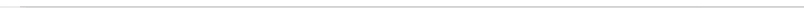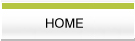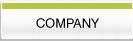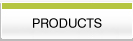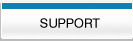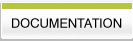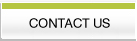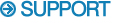Stacked Plots with Negative Data Doing Stacked Plots with Negative Data. Often a user may have a series of spectra (such as from a T1 experiment) that they wish to do a stacked plot of. However there is no easy way to set the offset for this type of plot so that negative peaks will be displayed correctly. Thus some spectra in the series (such as those from the short delay times) will tend to plot improperly with the negative peaks cut off at the lower edge of the plot. The following procedure should serve as a workaround for this problem. First make a copy of the matrix that you want to do the stack plot of. Then open this new matrix and set it to be writable. You would then run a short macro such as this: c**stack_offset.mac def datype 0 def datsiz &d1size loa 0 1 dr eva offset (-&smalpt) ty The offset is &smalpt for row 1 &d2size set &offset stb 1 loa 0 &row adb 1 ldb 1 sto 0 &row nex ex &return end This macro assumes that the first row in the matrix contains the most negative values. It loads this first row and uses the "smalpt" symbol which contains the smallest data value (most negative) to define an offset for the entire matrix. The macro then goes through the entire matrix row by row and adds in this offset to the data. All the data will then be positive and should produce a nice stacked plot. Note that the matrix is modified by this macro and you should only run this macro on a copy of the original data. The resulting matrix should then only be used for the stacked plot display. Note that if the most negative row is not the first row then you could modify the macro to look through the rows and use the most negative value that it finds. In this way it wouldn't matter what order the data was collected in or which row contained the most negative data. •   Back to FAQ's    •   Contact us www.FelixNMR.com © 2007